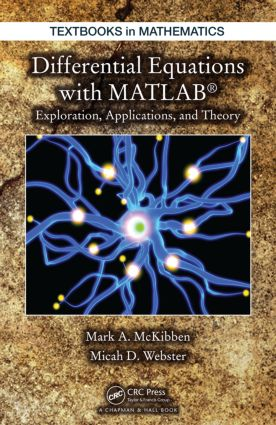# Differential Equations with MATLAB

## Exploration, Applications, and Theory, 1st Edition

Chapman and Hall/CRC

497 pages | 215 B/W Illus.

##### Purchasing Options:\$ = USD
Hardback: 9781466557079
pub: 2014-09-08
\$120.00
x
eBook (VitalSource) : 9780429097836
pub: 2014-09-08
from \$28.98

FREE Standard Shipping!

### Description

A unique textbook for an undergraduate course on mathematical modeling, Differential Equations with MATLAB: Exploration, Applications, and Theory provides students with an understanding of the practical and theoretical aspects of mathematical models involving ordinary and partial differential equations (ODEs and PDEs). The text presents a unifying picture inherent to the study and analysis of more than 20 distinct models spanning disciplines such as physics, engineering, and finance.

The first part of the book presents systems of linear ODEs. The text develops mathematical models from ten disparate fields, including pharmacokinetics, chemistry, classical mechanics, neural networks, physiology, and electrical circuits. Focusing on linear PDEs, the second part covers PDEs that arise in the mathematical modeling of phenomena in ten other areas, including heat conduction, wave propagation, fluid flow through fissured rocks, pattern formation, and financial mathematics.

The authors engage students by posing questions of all types throughout, including verifying details, proving conjectures of actual results, analyzing broad strokes that occur within the development of the theory, and applying the theory to specific models. The authors’ accessible style encourages students to actively work through the material and answer these questions. In addition, the extensive use of MATLAB® GUIs allows students to discover patterns and make conjectures.

### Reviews

"The purpose of this book is to illustrate the use of MATLAB in the study of several models involving ordinary and partial differential equations. It includes different disciplines such as physics, engineering and finance. It may be useful for engineers, physicists and applied mathematicians and also for advanced undergraduate (or beginning graduate) students who are interested in the utilization of MATLAB in differential equations. … The volume incorporates many figures and MATLAB exercises and many questions are raised throughout the text so that readers can do their own computer experiments."

—Antonio Canada Villar (Granada), writing in Zentralblatt MATH 1320 – 1

ORDINARY DIFFERENTIAL EQUATIONS

Welcome!

Introduction

This Book Is a Field Guide. What Does That Mean for YOU?

Mired in Jargon - A Quick Language Lesson!

Introducing MATLAB

A First Look at Some Elementary Mathematical Models

A Basic Analysis Toolbox

Some Basic Mathematical Shorthand

Set Algebra

Functions

The Space (R; j_j)

A Closer Look at Sequences in (R; j_j)

The Spaces (RN; k_kRN ) and (MN(R); k_kMN(R)

Calculus of RN-valued and MN(R)-valued Functions

Some Elementary ODEs

A First Wave of Mathematical Models

Newton's Law of Heating and Cooling-Revisited

Pharmocokinetics

Uniform Mixing Models

Combat! Nation in Balance

Springs and Electrical Circuits - The Same, But Different

Boom! - Chemical Kinetics

Going, Going, Gone! A Look at Projectile Motion

Shake, Rattle, Roll!

My Brain Hurts! A Look at Neural Networks

Breathe In, Breathe Out-A Respiratory Regulation Model

Finite-Dimensional Theory - Ground Zero: The Homogenous Case

Introducing the Homogenous Cauchy Problem (HCP)

Lessons Learned from a Special Case

Defining the Matrix Exponential

Putzer's Algorithm

Properties of eAt

The Homogenous Cauchy Problem: Well-Posedness

Higher-Order Linear ODEs

A Perturbed (HCP)

What Happens to Solutions of (HCP) as Time Goes On and On and On…?

Finite-Dimensional Theory - Next Step: The Non-Homogenous Case

Introducing…The Non-Homogenous Cauchy Problem (Non-CP)

Carefully Examining the One-Dimensional Version of (Non-CP)

Existence Theory for General (Non-CP)

Dealing with a Perturbed (Non-CP)

What Happens to Solutions of (Non-CP) as Time Goes On and On and On…?

A Second Wave of Mathematical Models-Now, with Nonlinear Interactions

Newton's Law of Heating and Cooling Subjected to Polynomial Effects

Pharmocokinetics with Concentration-Dependent Dosing

Springs with Nonlinear Restoring Forces

Circuits with Quadratic Resistors

Enyzme Catalysts

Projectile Motion-Revisited

Floor Displacement Model with Nonlinear Shock Absorbers

Finite-Dimensional Theory - Last Step: The Semi-Linear Case

Introducing the Even-More General Semi-Linear Cauchy Problem (Semi-CP)

New Challenges

Behind the Scenes: Issues and Resolutions Arising in the Study of (Semi-CP)

Lipschitz to the Rescue!

Gronwall's Lemma

The Existence and Uniqueness of a Mild Solution for (Semi-CP)

Dealing with a Perturbed (Semi-CP)

ABSTRACT ORDINARY DIFFERENTIAL EQUATIONS

Getting the Lay of a New Land

A Hot Example

The Hunt for a New Abstract Paradigm

A Small Dose of Functional Analysis

Three New Mathematical Models

Turning Up the Heat - Variants of the Heat Equation

Clay Consolidation and Seepage of Fluid through Fissured Rocks

The Classical Wave Equation and its Variants

An Informal Recap: A First Step toward Unification

Formulating a Theory for (A-HCP)

Introducing (A-HCP)

Defining eAt

Properties of eAt

The Abstract Homogeneous Cauchy Problem: Well-Posedness

A Brief Glimpse of Long-Term Behavior

The Next Wave of Mathematical Models - With Forcing

Turning Up the Heat - Variants of the Heat Equation

Seepage of Fluid through Fissured Rocks

The Classical Wave Equation and its Variants

Remaining Mathematical Models

Population Growth-Fisher's Equation

Zombie Apocalypse! - Epidemiological Models

How Did That Zebra Gets Its Stripes? - A First Look at Spatial Pattern Formation

Autocatalysis-Combustion!

Money, Money, Money - A Simple Financial Model

Formulating a Theory for (A-NonCP)

Introducing (A-NonCP)

Existence and Uniqueness of Solutions of (A-NonCP)

Dealing with a Perturbed (A-NonCP)

Long-Term Behavior

A Final Wave of Models - Accounting for Semilinear Effects

Turning Up the Heat - Semi-Linear Variants of the Heat Equation

The Classical Wave Equation with Semilinear Forcing

Population Growth-Fisher's Equation

Zombie Apocalypse! - Epidemiological Models

How Did That Zebra Gets Its Stripes? - A First Look at Spatial Pattern Formation

Autocatalysis-Combustion!

Epilogue

Appendix

Bibliography

Index

### Subject Categories

##### BISAC Subject Codes/Headings:
MAT000000
MATHEMATICS / General
MAT003000
MATHEMATICS / Applied
MAT007000
MATHEMATICS / Differential Equations